# reactance

(redirected from inductive resistance)
Also found in: Dictionary, Thesaurus, Medical.
Related to inductive resistance: Inductive reactance

## reactance:

see impedanceimpedance,
in electricity, measure in ohms of the degree to which an electric circuit resists the flow of electric current when a voltage is impressed across its terminals.
.

## Reactance

The imaginary part of the impedance of an alternating-current circuit.

The impedance Z of an alternating current circuit is a complex number given by Eq. (1).

(1)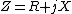(2)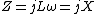(3)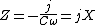The imaginary part X is the reactance. The units of reactance, like those of impedance, are ohms. Reactance may be positive or negative. For example, the impedance of an inductor L at frequency &ohgr; is given by Eq. (2), so X is positive. The impedance of a capacitor C is given by Eq. (3), so X is negative.

The reactance of a circuit may depend on both the resistors and the inductors or capacitors in the circuit. For example, the circuit in the illustration has admittance [Eq. (4)] and impedance [Eq. (5)], so that the reactance [Eq. (6)], depends on both the capacitor C and the resistor R. (4)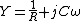(5)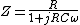(6)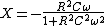See Admittance, Electrical impedance

McGraw-Hill Concise Encyclopedia of Physics. © 2002 by The McGraw-Hill Companies, Inc.
The following article is from The Great Soviet Encyclopedia (1979). It might be outdated or ideologically biased.

## Reactance

in electricity, a quantity characterizing the opposition presented to an alternating current by the capacitance and inductance of a circuit or part of a circuit. Reactance is measured in ohms.

In the case of a sinusoidal current in a circuit where inductive and capacitive circuit elements are connected in series, the reactance x can be expressed as the difference between the inductive and capacitive reactances: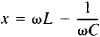Here, to is the angular frequency of the current, L is the inductance of the circuit, and C is the capacitance of the circuit. Reactance is equal to the ratio of the amplitude of the voltage on the terminals of a circuit having little resistance and the amplitude of the current through the circuit. When an alternating current flows in a circuit having only reactance, energy is transferred from the current source to the electric or magnetic field produced, respectively, by the capacitive or inductive circuit element and then back to the current source; the average power during a period is equal to zero. The presence of reactance in a circuit causes a phase difference between the voltage and the current.

When the current in a circuit is nonsinusoidal, the reactance is different for the individual harmonic components of the current.

## reactance

[rē′ak·təns]
(electricity)
The imaginary part of the impedance of an alternating-current circuit.
McGraw-Hill Dictionary of Scientific & Technical Terms, 6E, Copyright © 2003 by The McGraw-Hill Companies, Inc.

## reactance

1. the opposition to the flow of alternating current by the capacitance or inductance of an electrical circuit; the imaginary part of the impedance Z, Z = R + iX, where R is the resistance, i = &#221A--1, and X is the reactance. It is expressed in ohms
2. the opposition to the flow of an acoustic or mechanical vibration, usually due to inertia or stiffness. It is the magnitude of the imaginary part of the acoustic or mechanical impedance
Collins Discovery Encyclopedia, 1st edition © HarperCollins Publishers 2005
References in periodicals archive ?
At [f*.sub.n] > 5, the value of the internal inductive resistance of the trolley lines is small compared with the external inductive resistance, therefore, with enough accuracy for practice, it is possible to use the relation:
Calculation of the inductive resistance of the equivalent model of the current limiter in nominal mode is performed on the basis of the calculation of the magnetic field and is shown in Table 3.
Elements of the blocks of the Jacobi matrix are the differential inductive resistances of the motor's circuits, which are determined by calculating the magnetic circuit of the SSM in accordance with the accepted model .

Site: Follow: Share:
Open / Close